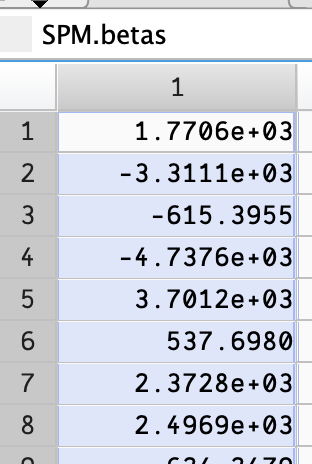# Crazy high beta/percent signal change values

I’m trying to extract PSC from several ROIs.
The values I’m getting are in the hundreds:

subject event event_name psc
1 1 IntactSame1 233.958786
1 2 IntactSame2 -539.485409
1 3 IntactSame3 312.277228
1 4 IntactSame4 78.178879
1 5 IntactAlt1 192.514225

I’m using marsbar code per their suggestion:

``````
% Make marsbar design object
D  = mardo(spm_name);

% ReML errors, the following uses an alternate method.
% See: https://marsbar-toolbox.github.io/faq.html#i-get-errors-using-the-spm-reml-estimation-for-fmri-designs-can-i-try-something-else
D = autocorr(D, 'fmristat', 2);

% Make marsbar ROI object
R  = maroi(roi_filename);
% Fetch data into marsbar data object
Y  = get_marsy(R, D, 'mean');
% Get contrasts from original design
xCon = get_contrasts(D);
% Estimate design on ROI data
E = estimate(D, Y);
% Put contrasts from original design back into design object
E = set_contrasts(E, xCon);

% Get definitions of all events in model
[e_specs, e_names] = event_specs(E);
n_events = size(e_specs, 2);
dur = 0;
% Return percent signal estimate for all events in design
for e_s = 1:n_events
pct_ev(ndx,e_s) = event_signal(E, e_specs(:,e_s), dur);

fprintf(psc_file, '%d,%d,"%s",%f\n', ndx, e_s, char(e_names(e_s)), pct_ev(ndx, e_s));
...
``````

When I step into `event_signal` I see that the extreme values are coming from the beta weights.

``````...
e_s_l = size(e_spec, 2);
s     = 0;
s_mus = block_means(D);
SPM   = des_struct(D);
for e_i = 1:e_s_l
es    = e_spec(:, e_i);
ss    = es(1);
Yh    = event_fitted(D, es, dur);
d     = pr_ev_diff(Yh, diff_func, varargin{:});
s     = s + d ./ s_mus(ss,:);
end
s = s / e_s_l * 100;
``````

I can set a breakpoint on the `for` line, and see that `SPM.betas` has very large values.Contrasts, etc. here in this gist.

The only thing that’s unusual is I have five fixation conditions, one for each type of stimulus presented, but use -0.2 for each of those. This could be wrong. Each set of the video stimuli for each condition are presented four times, so each is weighted 0.25 in the contrasts.
So stimuli presentations and fixations look like:
`A A A A Afix`
`B B B B Bfix`
`A B A B ABfix`

Edit: Changed to use a single Fixation, and beta values are still very high. The fixation values (rows 12 and 24 here) seem to be several times less than the stimuli beta values.

I’m at a loss, and would appreciate any suggestions.